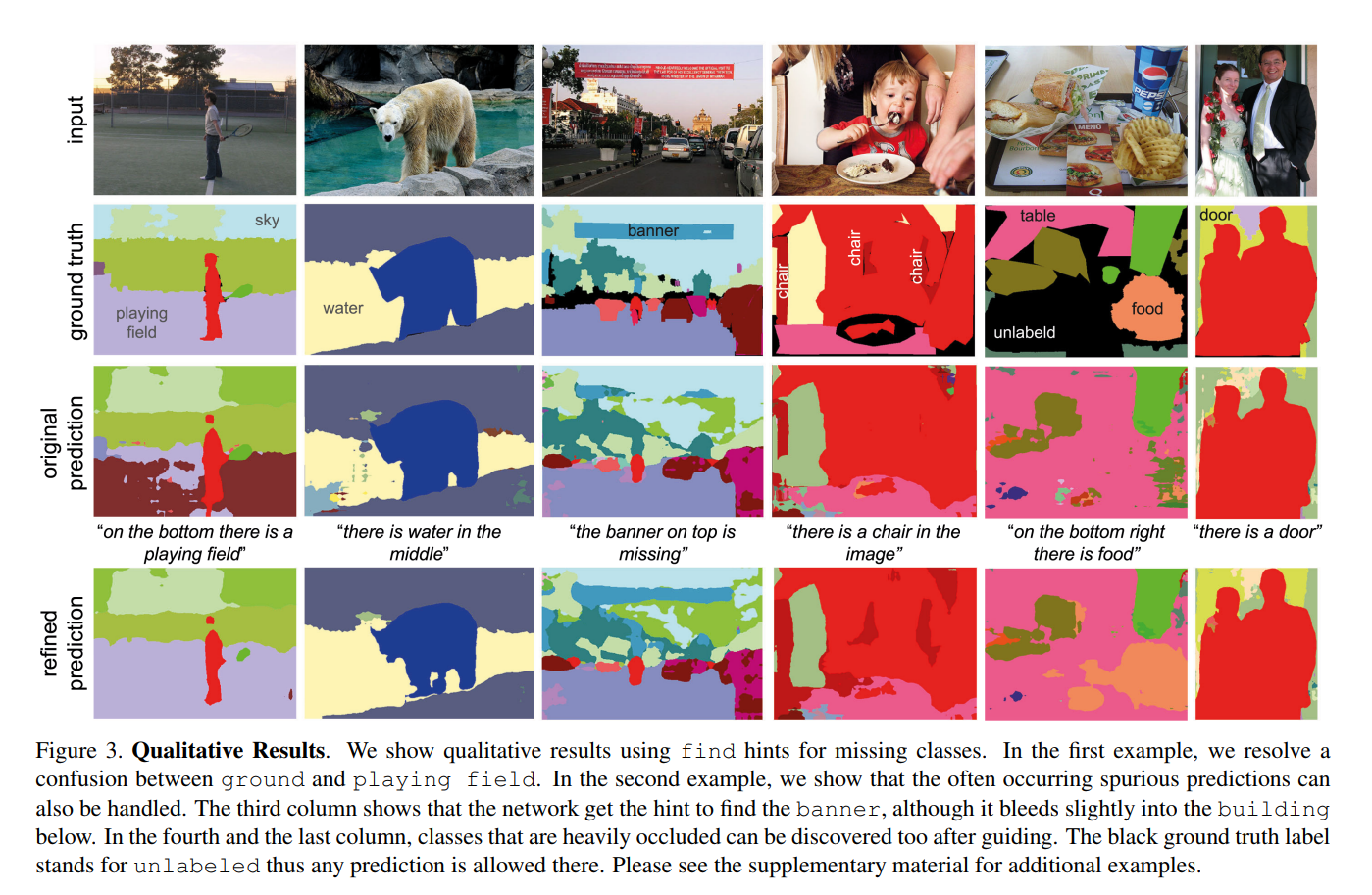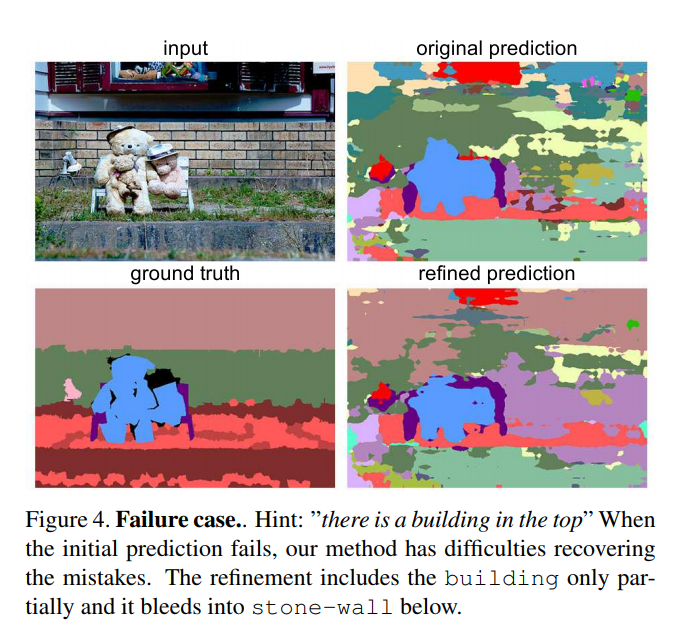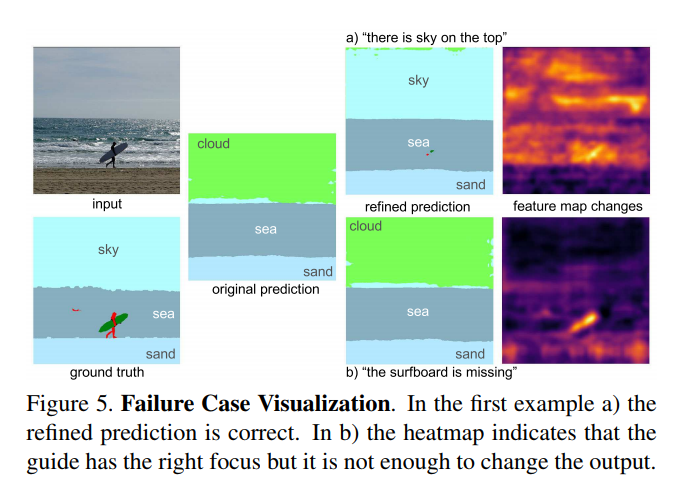## Summary

A “guiding block” is added inside a CNN (at the “smallest” encoding layer) that uses text hints to modify feature maps in order to refine the CNN’s prediction.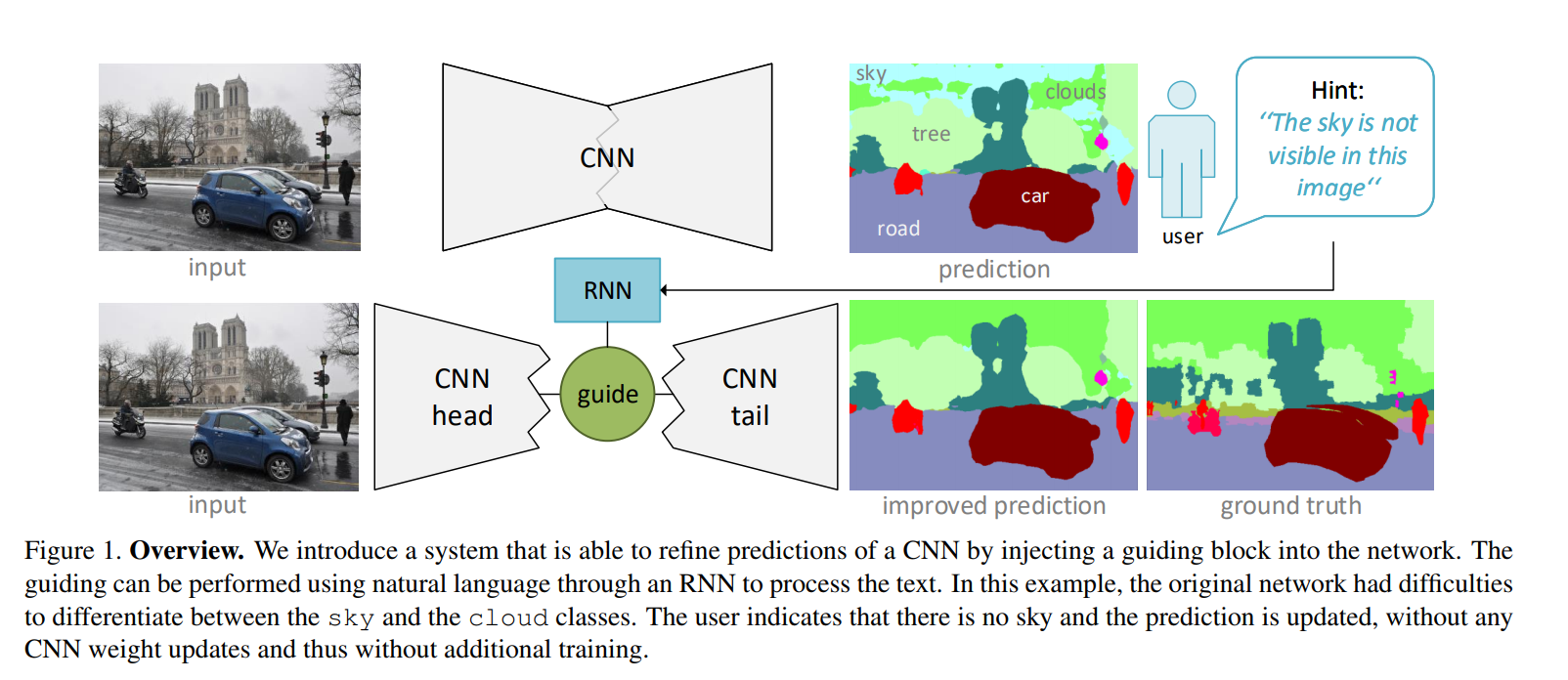### Guiding block

In order to modify activation map $$A \in \mathbb{R}^{H \times W \times C}$$, an RNN reads a sentence (parsed into a word embedding), and the last RNN state is fed to a dense layer that predicts:

• Channel re-weighting vector $$\gamma^{(s)} \in \mathbb{R}^C$$ and bias $$\gamma^{(b)} \in \mathbb{R}^C$$
• Spatial re-weighting vectors $$\alpha \in \mathbb{R}^H$$ and $$\beta \in \mathbb{R}^W$$

Thus, a single element of the modified feature map is: $$A^{\prime}_{h,w,c} = (1 + \alpha_h + \beta_w + \gamma^{(s)}_c) A_{h,w,c} + \gamma^{(b)}_c$$

This way, the number of parameters is much lower than a fully connected transformation. For example, for $$32 \times 32 \times 1024$$ activation maps, instead of $$\approx$$ 1 million parameters $$( H \times W \times C )$$, only 1088 $$( H + W + C )$$ are needed.

### Hint generation algorithm

An automatic hint generation algorithm was developed to generate hints that are useful for the model.

Using the ground truth and the predicted segmentation, a “query” is created based on a few criteria such as missing semantic classes, noise in the prediction or wrongly predicted pixels that should be replaced.

To do so, the image is divided in a coarse grid, and each cell is evaluated for missing or mistaken classes. Then, a class is selected from the possible choices and a query is generated based on the position in the grid.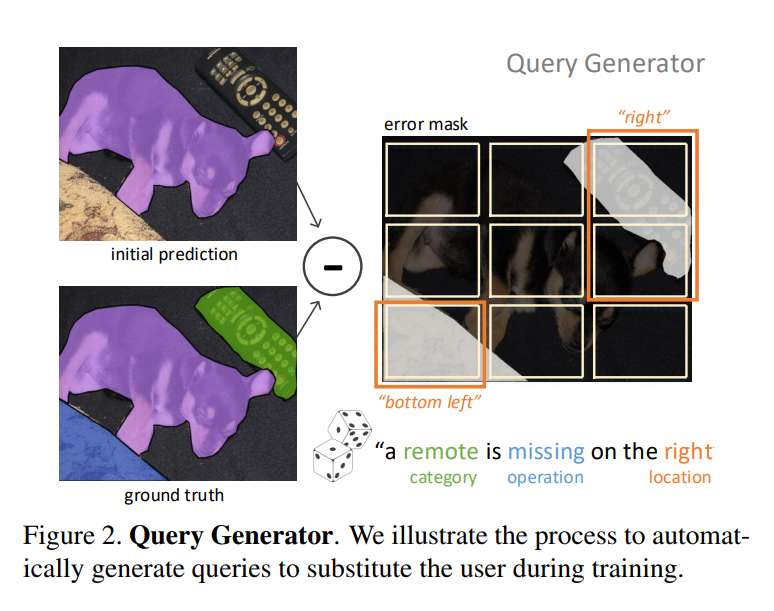## Experiments

Various experiments were done on PascalVOC (2012) and MSCOCO-Stuff (2014), that evaluate where the guiding block should be placed, how many hints are needed, how complex should the hints be, etc.